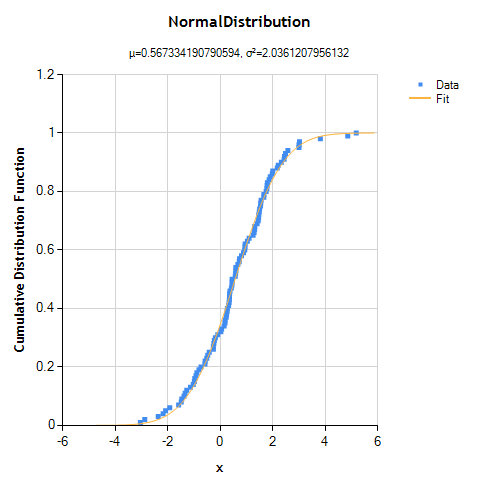PDF C#

Distribution Fitting DemoA customer recently asked how to fit a normal (Gaussian) distribution to a vector of experimental data. Here's a demonstration of how to do it. Let's start by creating a data set: 100 values drawn from a normal distribution with known parameters (mean = 0.5, variance = 2.0). int n = 100; double mean = .5; double variance = 2.0; var data = new DoubleVector( n, new RandGenNormal( mean, variance ) )...